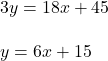## What is the slope of a line parallel to the line whose equation is 18x-3y=-4518x−3y=−45. Fully reduce your answer

Question

What is the slope of a line parallel to the line whose equation is 18x-3y=-4518x−3y=−45. Fully reduce your answer

in progress 0
2 months 2021-07-25T04:44:11+00:00 1 Answers 0 views 0

1. Given :

A line 18x – 3y = -45 .

To Find :

The slope of a line parallel to the given line.

Solution :

Simplifying given equation in form of :

y = mx + c.So, slope of above equation is 6.

Therefore, the slope of a line parallel to the given line is 6.

Hence, this is the required solution.